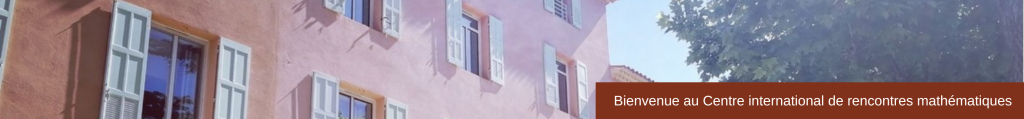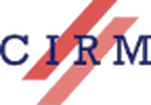CONFERENCE
Effective Analysis: Foundations, Implementations, Certification
January 11 – 15, 2016
 Effective analysis is the foundation of both symbolic and numerical algorithms which compute properties of continuous objects and of their implementation in mathematical software. This workshop aims to bring together researchers from different areas, interested in effective methods in analysis and geometry, and by the design and implementation of efficient and certified algorithms. Its objective is to stimulate discussions between communities that do not so often meet in traditional venues and to foster interactions between their different but complementary perspectives, in the tradition of the meetings of the MAP community. It will address perspectives from proof theory, type theory, constructive analysis, machine-checked mathematics, implementation of real/float/interval arithmetic, computer algebra systems, including those based on new paradigms like quantum computation. Scientific Committee University of Gothenburg)Assia Mahboubi (Inria Saclay)Marie-Françoise Roy (Université Rennes 1)University of Verona)Bas Spitters (Aarhus University) Organizing Committee Assia Mahboubi (Inria Saclay)Peter Schuster (University of Verona)Bas Spitters (Aarhus University) Speakers Andrej Bauer (University of Ljubljana) Tutorial on Type theory Ulrich Berger (Swansea University) Constructive logic for concurrent real number computation Sylvie Boldo (INRIA Saclay) Formal verification of numerical analysis programs Pieter Collins (Maastricht University) Implementing Logic and Real Arithmetic Eva Darulova (Max Planck Institute for Software Systems) Programming with Numerical Uncertainties Boris Djalal (INRIA) Newton sums for an effective formalization of algebraic numbers Fabian Immler (TUM Munich) Verified Numerics for ODEs in Isabelle/HOL Michal Konečný (Aston University) Exact Real Number Computation in AERN Catherine Lelay (Institute for Advanced Study) A new approach to formalize real numbers in the UniMath library Rob Lewis (Carnegie Mellon University) Algebra and Analysis in the Lean Theorem Prover Henri Lombardi (Université de Franche-Comté) Towards a constructive theory of O-minimal structures Victor Magron (Verimag Grenoble) Certified Roundoff Error Bounds Using Semidefinite Programming and Formal Floating Point Arithmetic Erik Martin-Dorel (Université Paul Sabatier Toulouse) CoqInterval: A Toolbox for Proving Non-linear Univariate Inequalities in Coq Norbert Müller (University of Trier) Wrapping in Exact Real Arithmetic Iosif Petrakis (University of Munich) Bishop’s Stone-Weierstrass theorem for compact metric spaces revisited Sebastian Posur (Aachen University) Category theory as a foundation for algorithms and programming in computer algebra Egbert Rijke (Carnegie Mellon University) Localizations at omega-compact types as sequential colimits Monika Seisenberger (Swansea University) Verification of Discrete and Real-timed \\ Railway Control Systems Peter Selinger (Dalhousie University) Combining numerical and number-theoretic methods to solve unitary approximation problems in quantum computing Bas Spitters (Aarhus University) Cubical sets as a classifying topos Laurent Théry (Inria – Sophia Antipolis) Proof and Computation Geum Young Hee (Dankook University) Basins of attraction for optimal third-order multiple-root finders